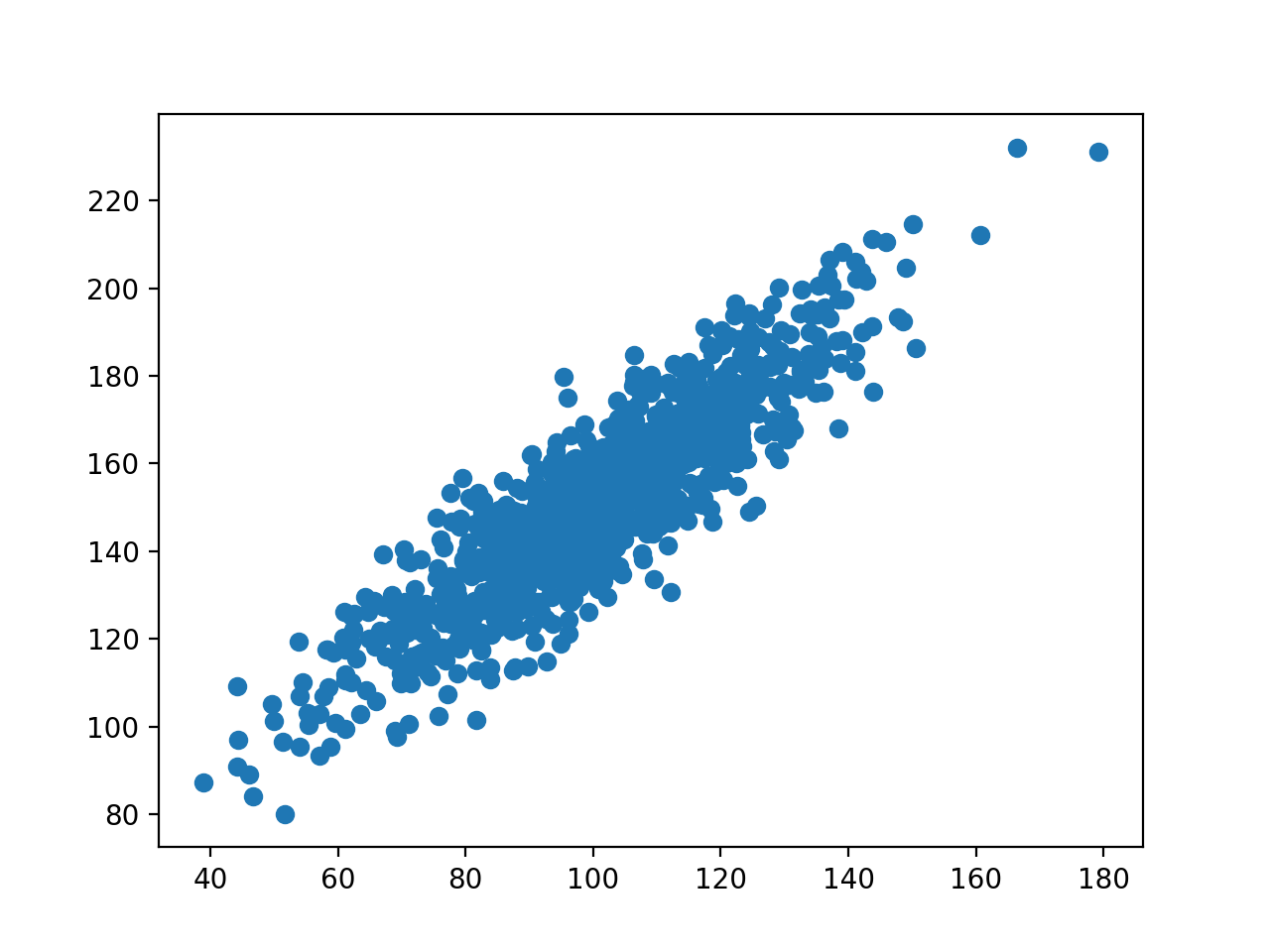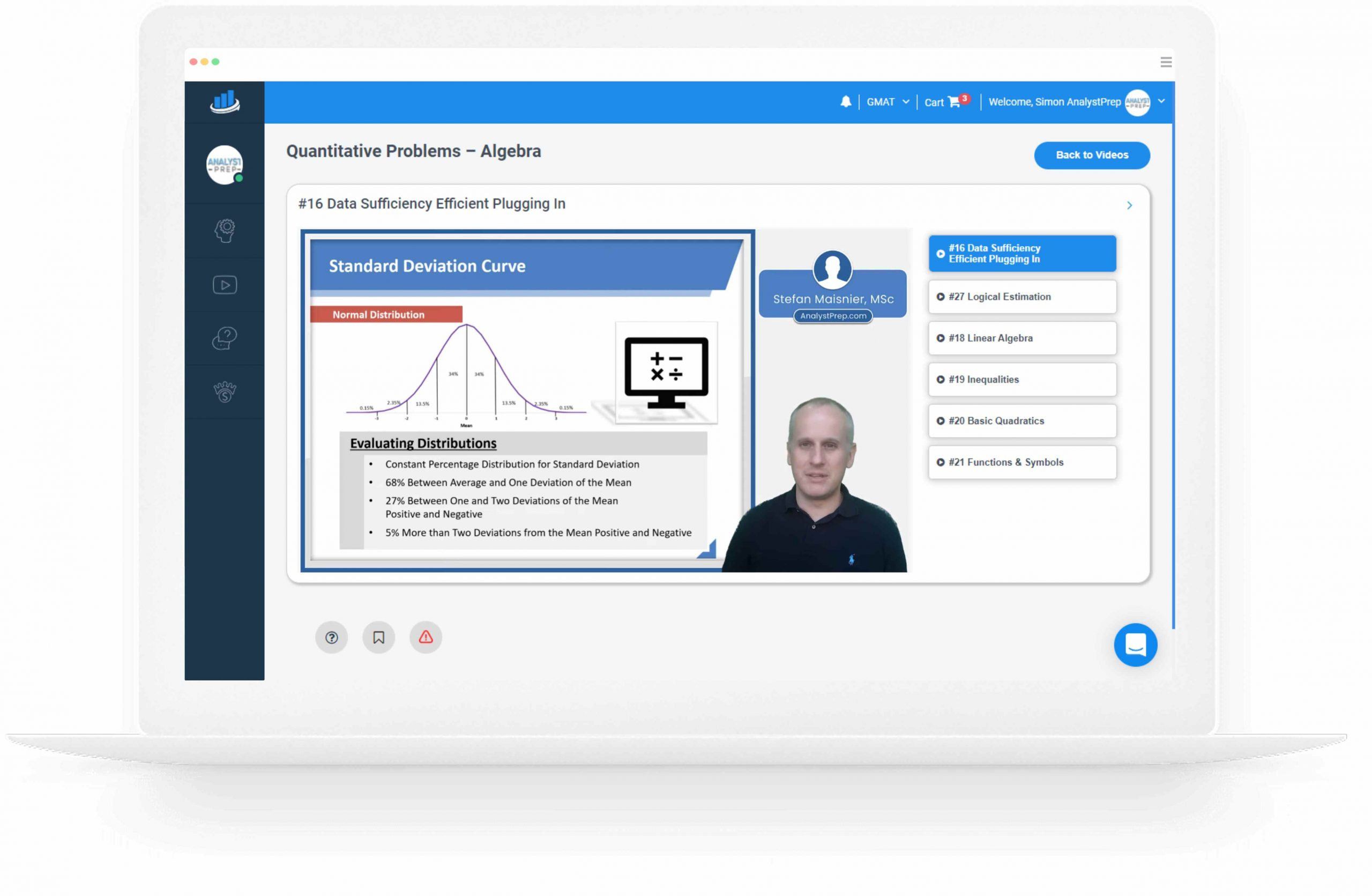# Covariance

The covariance is a measure of the degree of co-movement between two random variables. For instance, we could be interested in the degree of co-movement between the rate of interest and the rate of inflation.

• X = interest rate
• Y = inflation

The general formula used to calculate the covariance between two random variables, X and Y, is:

$$COV\ [X,\ Y] =E[(X-E[X]) (Y-E[Y])]$$

The covariance between two random variables can be positive, negative, or zero.

• A positive number indicates co-movement (i.e., the variables tend to move in the same direction);
• A value of zero indicates no relationship; and
• A negative value shows that the variables move in opposite directions.

Moreover, if variables are independent, their covariance is zero, i.e.,

$$COV\left(X,Y\right)=E\left(XY\right)-E\left(X\right)E\left(Y\right)=0$$

Covariances can be represented in a tabular format in a covariance matrix as follows:

 Asset A B C A Cov(A, A) Cov(A, B) Cov(A, C) B Cov(B, A) Cov(B, B) Cov(B, C) C Cov(C, A) Cov(C, B) Cov(C, C)
• The off-diagonal terms represent variances since Cov(C, C) = Var(C).
• A two-asset portfolio would have a similar 2 × 2 matrix.
• A correlation matrix can also be created to represent the correlations between various assets in a large portfolio.

#### Example 1: Calculating the covariance of a portfolio of two assets

A portfolio comprises two stocks – 1 and 2. The returns for the last 5 years are as follow:

Stock 1: 5%; 4.5%; 4.8%; 5.5%; 6%.

Stock 2: 6%; 6.2%; 5.7%; 6.1%; 6.5%.

Compute the covariance.

#### Solution

Step 1: We calculate the weighted sum of each stock to get the expected return on Stock 1 and Stock 2

$$E(R_1) = \frac{5\%+4.5\%+4.8\%+5.5\%+ 6\%}{5} = 5.2\%$$

$$E(R_2) = \frac{6\%+ 6.2\%+ 5.7\%+ 6.1\%+ 6.5\%}{5} = 6.1\%$$

Step 2: We subtract each year’s return from the expected return to obtain [R1-E(R1)] and [R2-E(R2)] as follows:

 Year R1 R2 [R1-E(R1)] [R2-E(R2)] 1 5% 6% -0.2% -0.1% 2 4.5% 6.2% -0.7% 0.1% 3 4.8% 5.7% -0.4% -0.4% 4 5.5% 6.1% 0.3% 0% 5 6% 6.5% 0.8% 0.4%

Step 3: We multiply the values obtained in Step 2 and we divide by the number of observations to get a mean observation.

$$Cov\left(R_1,R_2\right)=E\left[R_1-E\left(R_1\right)\right]\left[R_2-E\left(R_2\right)\right]$$
$$=\frac{(-0.2\%\times-0.1\%)+(-0.7\%\times0.1\%)+(-0.4\%\times-0.4\%)+(0.3\%\times0\%)+(0.8\%\times0.4\%)}{5}$$
$$=\frac{0.000043}{5}=0.0000086$$

#### Example 2: Covariance

Consider a set of a well-diversified portfolios X and Y. Suppose that the mean returns for X and Y are 6.12 and 7.04, respectively, what is the covariance between these portfolios?

#### Solution

Recall,

$$COV\left(X,Y\right)=E\left(XY\right)-E\left(X\right)E\left(Y\right)$$, for independent variables,

$$E\left(XY\right)=E\left(X\right)E\left(Y\right)=6.12\times7.04=43.0848$$

Which implies that,

$$COV\left(X,Y\right)=E\left(XY\right)-E\left(X\right)E\left(Y\right)=43.0848-6.12\left(7.04\right)=0$$

# Correlation

Correlation is the ratio of the covariance between two random variables and the product of their two standard deviations i.e.

$$\text{Correlation}\ (X_1,X_2\ )=\frac{Cov(X_1,X_2\ )}{Standard\ deviation\ (X_1\ )\times Standard\ deviation\ (X_2\ )}$$

Correlation measures the strength of the linear relationship between two variables. While the covariance can take on any value between negative infinity and positive infinity, the correlation is always a value between -1 and +1.

A correlation of -1 indicates a perfect inverse relationship (i.e. a unit change in one means that the other will have a unit change in the opposite direction). Secondly, a correlation of +1 indicates a perfect linear relationship (i.e. the two variables move in the same direction with the unit changes being equal). Finally, a correlation of zero implies that there is no linear relationship between the variables.

#### Example: Calculating the covariance

We anticipate that there is a 15% chance that next year’s stock returns for ABC Corp will be 6%, a 60% probability that they will be 8% and a 25% probability that they will be 10%. We already know the expected value of returns is 8.2% and the standard deviation is 1.249%.

We also anticipate that the same probabilities and states are associated with a 4% return for XYZ Corp, a 5% return, and a 5.5% return. The expected value of returns is then 4.975 and the standard deviation is 0.46%.

\begin{align*} \text{Covariance}, \text{cov}(\text R_{ \text{ABC}},\text R_{ \text{XYZ}}) & = 0.15(0.06 – 0.082)(0.04 – 0.04975) \\ & + 0.6(0.08 – 0.082)(0.05 – 0.04975) \\ & + 0.25(0.10 – 0.082)(0.055 – 0.04975) \\ & = 0.0000561 \\ \end{align*}

$$\text{Correlation}\ (R_i,R_j\ )=\frac{Cov(R_i,R_j\ )}{(Standard\ deviation\ (R_i\ )\times Standard\ deviation\ (R_j\ ))}$$

Thus,

$$\text{Correlation}=0.0000561\left(0.01249\times0.0046\right)=0.976$$

Interpretation: The correlation between the returns of the two companies is very strong (almost +1) and the returns move linearly in the same direction.

All 3 Levels of the CFA Exam – Complete Course offered by AnalystPrep

# How does Correlation Impact Portfolio Risk?

As mentioned earlier, correlation ranges from -1 to +1

• +1 = returns are perfectly positively correlated.
• 0 = returns of two assets are not correlated.
• -1 = returns are perfectly negatively correlated.
• What happens to portfolio risk (in a portfolio of two risky assets) when the two assets are perfectly correlated?
• Risk is unaffected; no benefit of diversification

In conclusion, using negatively correlated investments to form a portfolio helps to reduce the overall volatility of the portfolio.

Shop CFA® Exam Prep

Offered by AnalystPrepLevel I
Level II
Level III
All Three Levels
Featured Shop FRM® Exam PrepFRM Part I
FRM Part II
FRM Part I & Part II
Learn with Us

Subscribe to our newsletter and keep up with the latest and greatest tips for success

Shop Actuarial Exams PrepExam P (Probability)
Exam FM (Financial Mathematics)
Exams P & FM
Shop GMAT® Exam PrepComplete Course
Shop Executive Assessment® Exam PrepComplete Course